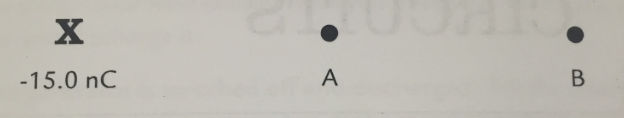# Problem: Suppose you have a fixed charge of -15.0 nC at point X. Points A and B are at distances of 20.0 cm and 40.0 cm from X as shown. Calculate the following:a) the potential at points A and Bb) the potential difference between A and Bc) the potential energy of a proton at A and Bd) the speed at point B of a proton that was moving to the right at point A with a speed of 4.0 × 105 m/se) the speed at point A of a proton that was moving to the left at point B with a speed of 1.0 × 105 m/s.

###### FREE Expert Solution

Potential, V:

$\overline{){\mathbf{V}}{\mathbf{=}}{\mathbf{k}}\frac{\mathbf{q}}{\mathbf{r}}}$

(a)

VA = (9.0 × 109)[(- 15.0 × 10-9)/(20.0 × 10-2)] = - 675 V

VB =  (9.0 × 109)[(- 15.0 × 10-9)/(40.0 × 10-2)] = - 338 V

100% (147 ratings)###### Problem Details

Suppose you have a fixed charge of -15.0 nC at point X. Points A and B are at distances of 20.0 cm and 40.0 cm from X as shown.Calculate the following:

a) the potential at points A and B

b) the potential difference between A and B

c) the potential energy of a proton at A and B

d) the speed at point B of a proton that was moving to the right at point A with a speed of 4.0 × 105 m/s

e) the speed at point A of a proton that was moving to the left at point B with a speed of 1.0 × 105 m/s.